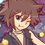# Inequalities (1st math Thailand POSN 2014)

Only use these following inequalities:

• A.M.-G.M.-H.M.
• Cauchy Schwarz
• Weighted A.M-G.M. with weight sum = 1

1.) Let $$a,b,c$$ be positive real numbers such that $\displaystyle \frac{\left(\displaystyle a+ \frac{1}{a}\right)\left(\displaystyle b+ \frac{1}{b}\right)\left(\displaystyle c+ \frac{1}{c}\right)}{abc} = 8$ Prove that $$abc \geq 1$$.

2.) Let $n$ be positive integers $n \geq 2$ and $x_{1},y_{1},x_{2},y_{2},...,x_{n},y_{n}$ be positive real numbers such that

$\sum\limits_{i = 1}^{n} x_{i} \geq \sum\limits_{i = 1}^{n} x_{i}y_{i}$

Prove that $\sum\limits_{i = 1}^{n} x_{i} \leq \sum\limits_{i = 1}^{n} \displaystyle \frac{x_{i}}{y_{i}}$

3.) Let $a,b,c$ be positive real numbers, prove that

$a^{3}+b^{3}+c^{3} \geq \frac{1}{4}(a+b+c)^{3} - 6abc$

4.) Let $a,b,c,d$ be positive real numbers and $36a+4b+4c+3d = 25$, prove that

$abcd(a+b+c+d)(ab^{1/2}c^{1/3}d^{1/4}) \leq \frac{1}{\sqrt{6}}(a^{4}b+b^{4}c+c^{4}d+d^{4}a)$

5.) Let $a\geq 2, b\geq 6, c \geq 12$, find and show the maximum value of

$\displaystyle \frac{bc\sqrt{a-2} + ca\sqrt{b-6} + ab\sqrt{c-12}}{abc}$

and find the conditions of $a,b,c$ to be maximum.

This is the part of Thailand 1st round math POSN problems.Note by Samuraiwarm Tsunayoshi
6 years, 8 months ago

This discussion board is a place to discuss our Daily Challenges and the math and science related to those challenges. Explanations are more than just a solution — they should explain the steps and thinking strategies that you used to obtain the solution. Comments should further the discussion of math and science.

When posting on Brilliant:

• Use the emojis to react to an explanation, whether you're congratulating a job well done , or just really confused .
• Ask specific questions about the challenge or the steps in somebody's explanation. Well-posed questions can add a lot to the discussion, but posting "I don't understand!" doesn't help anyone.
• Try to contribute something new to the discussion, whether it is an extension, generalization or other idea related to the challenge.
• Stay on topic — we're all here to learn more about math and science, not to hear about your favorite get-rich-quick scheme or current world events.

MarkdownAppears as
*italics* or _italics_ italics
**bold** or __bold__ bold
- bulleted- list
• bulleted
• list
1. numbered2. list
1. numbered
2. list
Note: you must add a full line of space before and after lists for them to show up correctly
paragraph 1paragraph 2

paragraph 1

paragraph 2

[example link](https://brilliant.org)example link
> This is a quote
This is a quote
    # I indented these lines
# 4 spaces, and now they show
# up as a code block.

print "hello world"
# I indented these lines
# 4 spaces, and now they show
# up as a code block.

print "hello world"
MathAppears as
Remember to wrap math in $$ ... $$ or $ ... $ to ensure proper formatting.
2 \times 3 $2 \times 3$
2^{34} $2^{34}$
a_{i-1} $a_{i-1}$
\frac{2}{3} $\frac{2}{3}$
\sqrt{2} $\sqrt{2}$
\sum_{i=1}^3 $\sum_{i=1}^3$
\sin \theta $\sin \theta$
\boxed{123} $\boxed{123}$

## Comments

Sort by:

Top Newest

For number 1, using the fact that a + (1/a) >= 2, this also applies for b and c. Hence, [a + (1/a)][b + (1/b)][c + (1/c)] >= 8. Hence, 8abc >= 8 and abc >= 1.

- 6 years, 8 months ago

Log in to reply

Yep, that's how I did it.

- 6 years, 8 months ago

Log in to reply

This is the easiest question because professor let everyone get at least 1 problem to bring some joy of solving the hardest topic of all.

- 6 years, 8 months ago

Log in to reply

first one is easy

- 6 years, 8 months ago

Log in to reply

Can someone tell me how to solve 4? I solved the rest.

- 5 years, 8 months ago

Log in to reply

Never mind. Just realised that 4 was quite easy.

- 5 years, 8 months ago

Log in to reply

For number 5, I'm trying to get the maximum value of 5/8sqrt(2) + 1/3 cube root(2)...

- 6 years, 8 months ago

Log in to reply

Trying #5...

$\displaystyle \frac{bc\sqrt{a-2} + ca\sqrt{b-6} + ab\sqrt{c-12}}{abc}$

$= \displaystyle \frac{\sqrt{a-2}}{a} + \frac{\sqrt{b-6}}{b} + \frac{\sqrt{c-12}}{c}$

Let $x = a-2, y = b-6, z = c-12$ such that $x,y,z \geq 0$.

The expression becomes $= \displaystyle \frac{\sqrt{x}}{x+2} + \frac{\sqrt{y}}{y+6} + \frac{\sqrt{z}}{z+12}$

By AM-GM; $\displaystyle \frac{\sqrt{x}}{x+2} \leq \frac{\sqrt{x}}{2\sqrt{2x}} = \frac{1}{2\sqrt{2}}$

Similarly, $\displaystyle \frac{\sqrt{y}}{y+3+3} \leq \frac{\sqrt{y}}{3\sqrt{y\times3^{2}}} = \frac{1}{3\times 3^{2/3}}$

$\displaystyle \frac{\sqrt{z}}{z+4+4+4} \leq \frac{\sqrt{z}}{4\sqrt{z\times4^{3}}} = \frac{1}{4\times 4^{3/4}}$

Sum all these up and we get

$\displaystyle \frac{bc\sqrt{a-2} + ca\sqrt{b-6} + ab\sqrt{c-12}}{abc} \leq \frac{1}{2\sqrt{2}}+\frac{1}{3\times 3^{2/3}}+\frac{1}{4\times 4^{3/4}}$ ~~~

Equality occurs if and only if $x=2,y=3,z=4$ which means $a=4,b=9,c=16$ ~~~

I don't have much time for doing these problems right now. I have to study math from POSN for whole October and I'm freaking busy reviewing lots of stuffs. heavy breathing

- 6 years, 8 months ago

Log in to reply

3rd one is preety easy. Do u still need it?

- 6 years, 7 months ago

Log in to reply

Yup I'm still stuck till now. =_="

- 6 years, 7 months ago

Log in to reply

×

Problem Loading...

Note Loading...

Set Loading...